NEET  >  Second Law of Motion

# Second Law of Motion Notes | Study Physics Class 11 - NEET

## Document Description: Second Law of Motion for NEET 2022 is part of Physics Class 11 preparation. The notes and questions for Second Law of Motion have been prepared according to the NEET exam syllabus. Information about Second Law of Motion covers topics like What is Newton’s Second Law of Motion?, What is a Net Force?, Application of Second Law&nbsp;, Newton’s Second Law Solved Examples and Second Law of Motion Example, for NEET 2022 Exam. Find important definitions, questions, notes, meanings, examples, exercises and tests below for Second Law of Motion.

Introduction of Second Law of Motion in English is available as part of our Physics Class 11 for NEET & Second Law of Motion in Hindi for Physics Class 11 course. Download more important topics related with notes, lectures and mock test series for NEET Exam by signing up for free. NEET: Second Law of Motion Notes | Study Physics Class 11 - NEET
 Table of contents What is Newton’s Second Law of Motion? What is a Net Force? Application of Second Law Newton’s Second Law Solved Examples
 1 Crore+ students have signed up on EduRev. Have you?

What is Newton’s Second Law of Motion?

Force is equal to the rate of change of momentum. For a constant mass, force equals mass times acceleration.

Newton’s second law of motion, unlike the first law of motion pertains to the behavior of objects for which all existing forces are unbalanced. The second law of motion is more quantitative and is used extensively to calculate what happens in situations involving a force.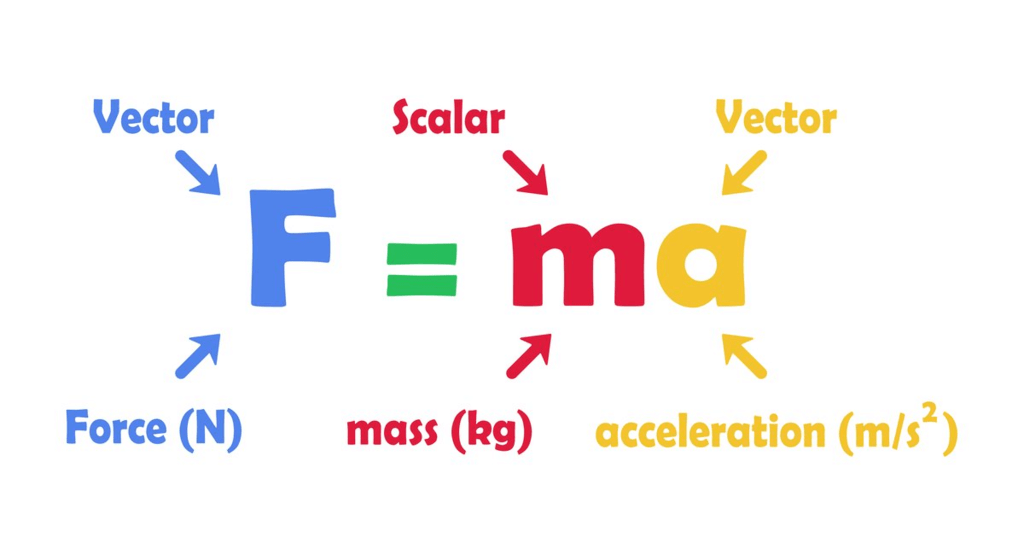Defining Newton’s Second Law of Motion

• Newton’s second law states that the acceleration of an object depends upon two variables – the net force acting on the object and the mass of the object.
• The acceleration of the body is directly proportional to the net force acting on the body and inversely proportional to the mass of the body.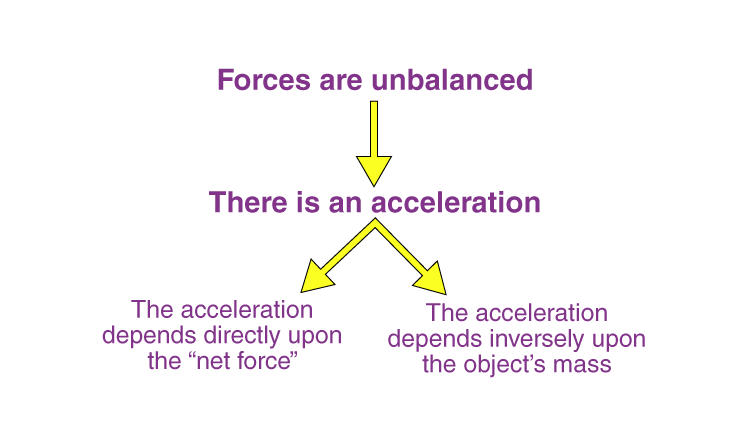• This means that as the force acting upon an object is increased, the acceleration of the object is increased. Likewise, as the mass of an object is increased, the acceleration of the object is decreased.

Newton’s second law can be formally stated as:

The acceleration of an object as produced by a net force is directly proportional to the magnitude of the net force, in the same direction as the net force, and inversely proportional to the mass of the object.

This statement is expressed in equation form as: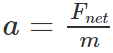The above equation can be rearranged to a familiar form as:

F = ma

Since force is a vector, Newton’s second law can be written as: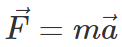The equation shows that the direction of the total acceleration vector points in the same direction as the net force vector.

Deriving Newton’s Second Law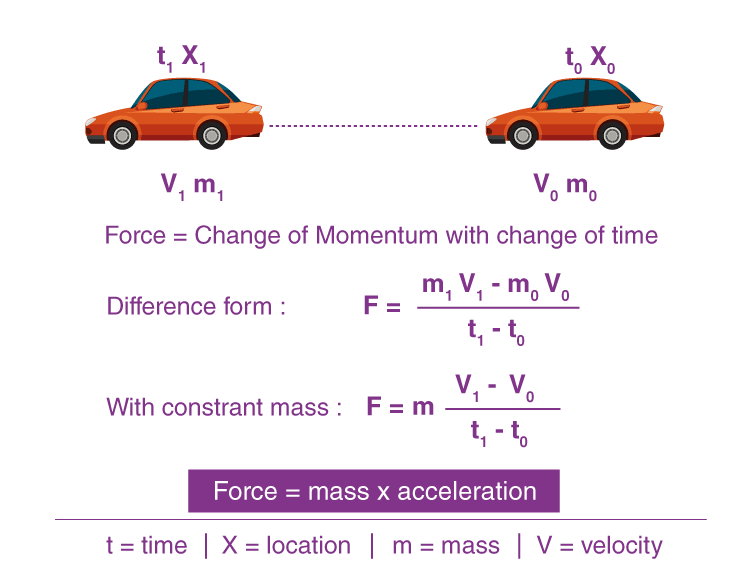1. For Changing Mass

• Let us assume that we have a car at a point (0) defined by location X0 and time t0. The car has a mass m0 and travels with a velocity v0
• After being subjected to a force F, the car moves to point 1 which is defined by location X1 and time t1. The mass and velocity of the car change during the travel to values m1 and v1.
•  Newton’s second law helps us determine the new values of m1 and v1 if we know the value of the acting force.
• Taking the difference between point 1 and point 0, we get an equation for the force acting on the car as follows: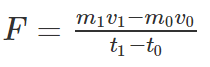Let us assume the mass to be constant. This assumption is good for a car because the only change in mass would be the fuel burned between point “1” and point “0”. The weight of the fuel is probably small relative to the weight of the rest of the car, especially if we only look at small changes in time. Meanwhile, if we were discussing the flight of a bottle rocket, then the mass does not remain constant and we can only look at changes in momentum.

2. For Constant Mass

• For a constant mass, Newton’s second law can be equated as follows: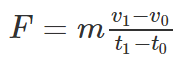• We know that acceleration is defined as the change in velocity divided by the change in time. The second law then reduces to a more familiar form as follows: F = ma
• The above equation tells us that an object will accelerate if it is subjected to an external force and the amount of force is directly proportional to the acceleration and inversely proportional to the mass of the object.

What is a Net Force?

• A net force ΣF is the sum of all forces acting on a body. More precisely, it is the vector sum of all forces acting on a body.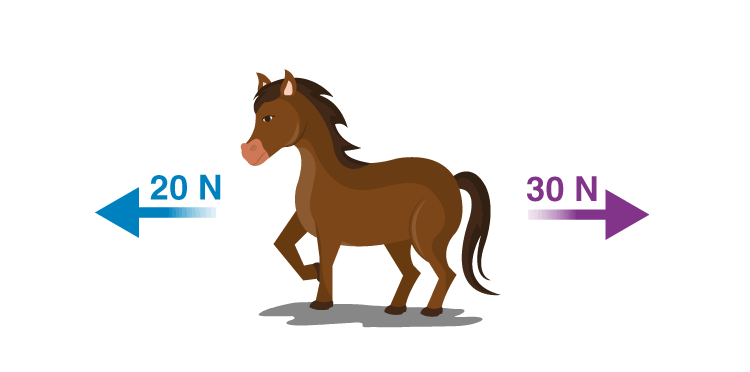• Consider two forces of magnitude 30 N and 20 N that are exerted to the right and left respectively on the horse shown in the picture.
• If we assume the rightward direction as positive, then the net force on the horse can be calculated as follows:
∑F = 30N − 20N = 10N
∑F = 10 N to the right

Application of Second Law

The application of the second law of motion can be seen in identifying the amount of force needed to make an object move or to make it stop.

Following are a few examples that we have listed to help you understand this point:

1. Kicking a ball
When we kick a ball we exert force in a specific direction, which is the direction in which it will travel. In addition, the stronger the ball is kicked, the stronger the force we put on it and the further away it will travel.
2. Pushing a cart
It is easier to push an empty cart in a supermarket than it is to push a loaded one. More mass requires more force to accelerate.
3. Two people walking
Among the two people walking, if one is heavier than the other then the one weighing heavier will walk slower because the acceleration of the person weighing lighter is greater.

Newton’s Second Law Solved Examples

Example 1: If there is a block of mass 2kg, and a force of 20 N is acting on it in the positive x-direction, and a force of 30 N in the negative x-direction, then what would be its acceleration?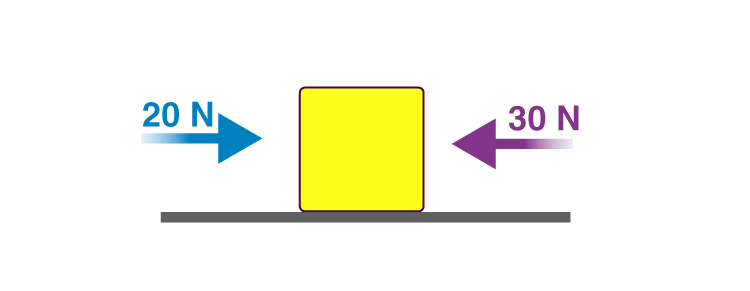Solution: To calculate its acceleration, we first have to calculate the net force acting on it.

Fnet  = 20 N – 30 N = -10 N
Mass = 2kg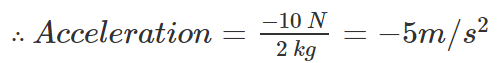The negative acceleration indicates that the block is slowing and its acceleration vector is moving in an opposite direction directed opposite to the direction of motion.

Example 2: How much horizontal net force is required to accelerate a 1000 kg car at 4 m/s2?
Solution: Newton’s 2nd Law relates an object’s mass, the net force on it, and its acceleration. Therefore, we can find the force as follows:

Fnet = ma

Substituting the values, we get
1000 kg × 4 m/s2 = 4000 N
Therefore, the horizontal net force is required to accelerate a 1000 kg car at 4 m/s2 is 4000 N.

Newton’s second law is applied in daily life to a great extent. For instance, in Formula One racing, the engineers try to keep the mass of cars as low as possible. Low mass will imply more acceleration, and the more the acceleration, the chances to win the race are higher.

The document Second Law of Motion Notes | Study Physics Class 11 - NEET is a part of the NEET Course Physics Class 11.
All you need of NEET at this link: NEET

## Physics Class 11

126 videos|449 docs|210 tests
 Use Code STAYHOME200 and get INR 200 additional OFF

## Physics Class 11

126 videos|449 docs|210 tests

### How to Prepare for NEET

Read our guide to prepare for NEET which is created by Toppers & the best Teachers

Track your progress, build streaks, highlight & save important lessons and more!

,

,

,

,

,

,

,

,

,

,

,

,

,

,

,

,

,

,

,

,

,

;